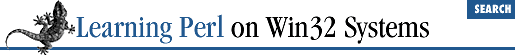home | O'Reilly's CD bookshelfs | FreeBSD | Linux | Cisco | Cisco Exam

### A.17 Chapter 18, CGI Programming

1. Here's one way to do it:

```use strict;
use CGI qw(:standard);
if(param()) {
my \$n1 = param('field1');
my \$n2 = param('field2');
my \$n3 = \$n2 + \$n1;
print p("\$n1 + \$n2 = <strong>\$n3</strong>\n");
} else {
print hr(), start_form();
print p("First Number:", textfield("field1"));
print p("Second Number:", textfield("field2"));
print end_form(), hr();
}
print end_html();```

We simply generate a form if there's no input with two textfields (using the ``` textfield()``` method). If there is input, we simply add the two fields together and print the result.

2. Here's one way to do it

```use strict;
use CGI qw(:standard);
print h1("Browser Detective"), hr();
my \$browser = \$ENV{'HTTP_USER_AGENT'};
\$_ = \$browser;
if (/msie/) {
msie(\$_);
} elsif (/mozilla/i) {
netscape(\$_);
} elsif (/lynx/i) {
lynx(\$_);
} else {
default(\$_);
}

print end_html();
sub msie{
print p("Internet Explorer: @_.  Good Choice\n");
}
sub netscape {
print p("Netscape: @_.  Good Choice\n");
}
sub lynx {
print p("Lynx: @_.  Shudder...");
}
sub default {
print p("What the heck is a @_?");
}```

The key here is checking the environment for the HTTP_USER_AGENT variable (line 5). Although this step isn't implemented by every server, many of them do set the variable. This method is a good way to generate content geared at the features of a particular browser. Note that we're just doing some basic string matching (case insensitive) to see what they're using (nothing too fancy). Experienced Perl programmers would probably prefer to write the string-matching section more along these lines:

```BROWSER:{
/msie/i    and do { msie(\$_), last BROWSER; };
/mozilla/i and do { netscape(\$_), last BROWSER; };
/lynx/i    and do { lynx(\$_), last BROWSER; };
default(\$_);
}```

However we haven't talked about this construct in this book. If you're interested, see Chapter 2 of Programming Perl for several other ways to emulate a switch construct.A.16 Chapter 17, Database ManipulationA.18 Chapter 19, OLE Automation